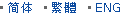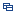| Recent Visit History | Join Audited Suppliers | Help | Region Guide
» » » » Nanjing Product List

136.

## Glass Meniscus Lens in Stock[Nov 20, 2020]

Meniscus lenses have one concave and one convex surface. For this lens type, the two curvatures are chosen to minimize the spherical aberration at one laser wavelength, hence ...

137.

## Silicon Glass Double Convex Lens[Nov 20, 2020]

Double convex lenses comprise two convex spherical surfaces, generally with the same radius of curvature. Bi convex lenses are used as magnifiers, condensing lenses and ...

138.

## Bk7 Optical Glass Plano Convex Focus Lens[Nov 20, 2020]

Plano convex lenses have one plano surface and one convex surface, they have a positive focal length. Plano convex lenses are widely used for focusing and collecting light in ...

139.

## Custom Made Plano Concave Lens Optical Glass[Nov 20, 2020]

Plano-concave lenses are thicker at the edge than in the center and flat on another surface. Plano concave lens (PCV) has negative focal length. So the lenses are used to bend ...

140.

## Bk7 Optical Glass Bi Convex Lens[Nov 20, 2020]

Double convex lenses have two outward curved surfaces, normally the two surfaces have same radius of curvature, with finely polished. Bi convex lenses have positive focal length, ...

141.

## Condenser Optical Glass Bi Convex Lens[Nov 20, 2020]

Double convex lenses have two outward curved surfaces, normally the two surfaces have same radius of curvature, with finely polished. Bi convex lenses have positive focal length, ...

142.

## Optical Glass Meniscus Lens for Binocular[Nov 20, 2020]

Meniscus lenses have one concave and one convex surface. For this lens type, the two curvatures are chosen to minimize the spherical aberration at one laser wavelength, hence ...

143.

## Focusing Lens Collimating Lens Optical Glass[Nov 20, 2020]

Double convex lenses have two outward curved surfaces, normally the two surfaces have same radius of curvature, with finely polished. Bi convex lenses have positive focal length, ...

144.

## Silicon Glass Bi Convex Lens[Nov 20, 2020]

Double convex lenses have two outward curved surfaces, normally the two surfaces have same radius of curvature, with finely polished. Bi convex lenses have positive focal length, ...

145.

## Condensing Lens Collimating Lens Glass Lens[Nov 20, 2020]

Double convex lenses have two outward curved surfaces, normally the two surfaces have same radius of curvature, with finely polished. Bi convex lenses have positive focal length, ...

146.

## Objective Optical Glass Bi Convex Lens[Nov 20, 2020]

Bi Convex lens has a positive focal length, and with two outward surfaces. The two surfaces are with same radius of curvature, thereby minimizing spherical aberration. Some ...

147.

## Optical Glass Bk7 Double Convex Focusing Lens[Nov 20, 2020]

Double convex lenses comprise two convex spherical surfaces, generally with the same radius of curvature. Bi convex lenses are used as magnifiers, condensing lenses and ...

148.

## Optical Glass Bk7 Biconvex Lens Ar Coated[Nov 20, 2020]

Double convex lenses have two outward curved surfaces, normally the two surfaces have same radius of curvature, with finely polished. Bi convex lenses have positive focal length, ...

149.

## Magnifier Bi Convex Lens Optical Glass[Nov 20, 2020]

Double convex lenses have two outward curved surfaces, normally the two surfaces have same radius of curvature, with finely polished. Bi convex lenses have positive focal length, ...

150.

## LED Lens Projector Lens Glass Lens[Nov 20, 2020]

Double convex lenses have two outward curved surfaces, normally the two surfaces have same radius of curvature, with finely polished. Bi convex lenses have positive focal length, ...

Hot Region Index: ABCDEFGHIJKLMNPQRSTUWXYZ